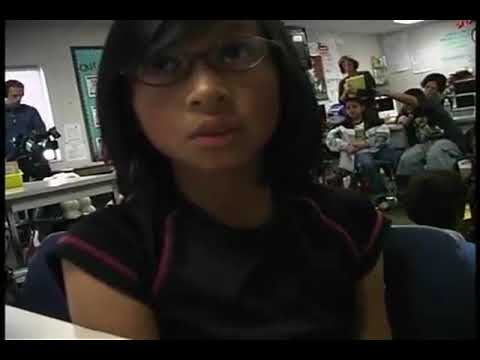Video

# Multiplying 3-digit by 1-digit (regrouping) (Full video)

Khan Academy

## Supporting grades: 4

Description: Learn to multiply a 3-digit number by a 1-digit number using regrouping. In this video, we will multiply 7x253. Created by Sal Khan. And then write the smaller number below it and align the place value, the 7. And I multiply it times each of the numbers up here, and I carry appropriately. What I would do in the standard method is I would write the 1 into 21 down here, but then carry the 2 to the tens place.

You must log inorsign upif you want to:*

*Teacher Advisor is 100% free.

### Other videos you might be interested in### Number Operations: Multiplication (Full video)

#### Inside Mathematics